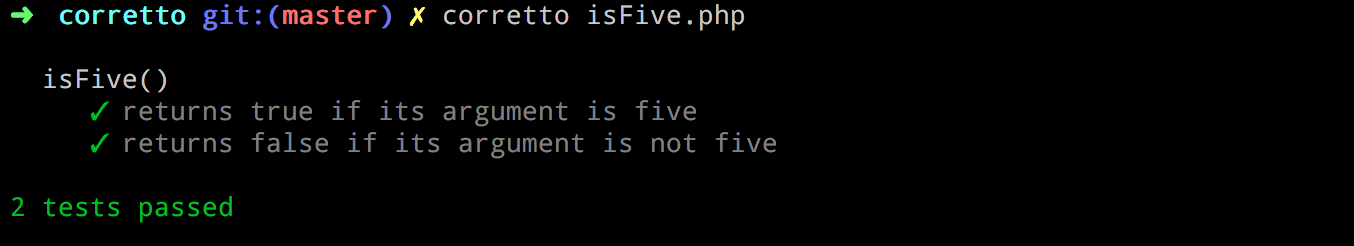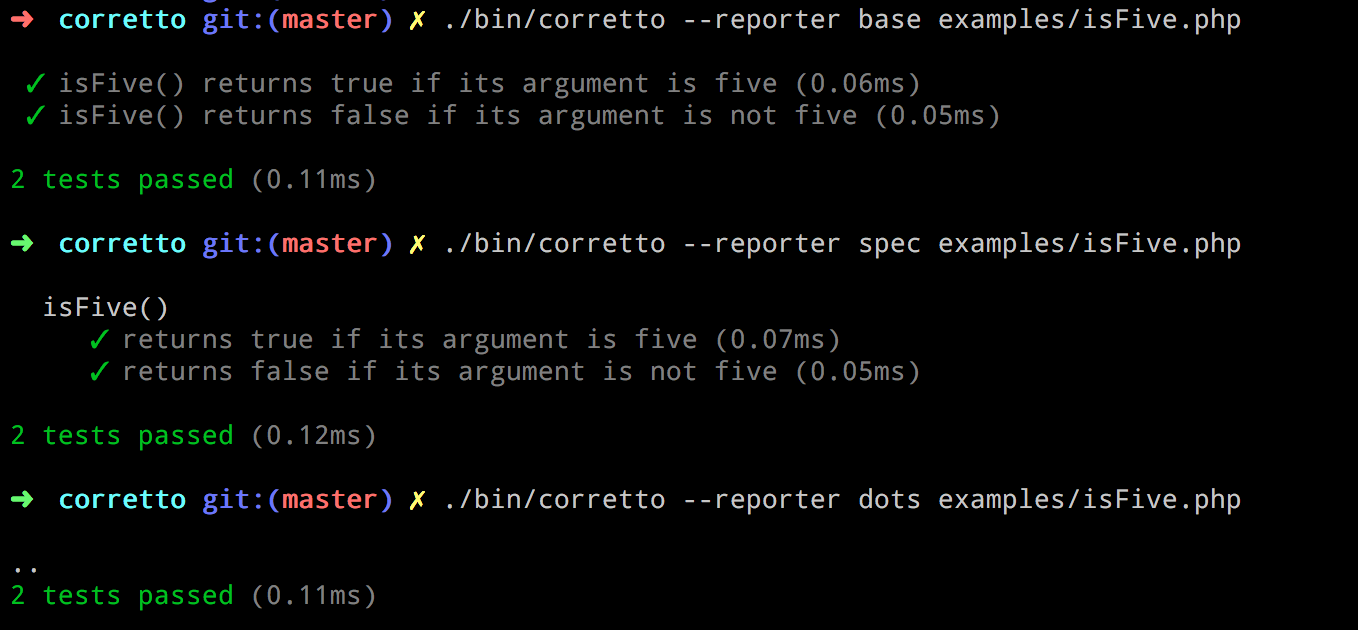## sirbrillig /corretto

A simple and expressive PHP test runner

##### Details

Homepage

Source

Issues

27

0

0

2

0

v1.4.0 2016-12-30 15:57 UTC

This package is auto-updated.

Last update: 2023-05-20 07:29:36 UTC

Corretto is a simple and expressive PHP test runner.

Modeled after mocha, Corretto provides a DSL (domain-specific language) that makes writing tests easy. No need for classes and long function names; just say what your code does in plain words. After all, wouldn't you rather spend most of your time working on code rather than writing tests?

<?php
use function Corretto\describe, Corretto\it, Corretto\expect;

describe( 'isFive()', function() {
it( 'returns true if its argument is five', function() {
expect( isFive( 5 ) )->toBeTrue();
} );

it( 'returns false if its argument is not five', function() {
expect( isFive( 6 ) )->toBeFalse();
} );
} );## Installing

First you'll need Composer. Then, in your project:

composer require --dev sirbrillig/corretto


Or, to install globally:

composer global require sirbrillig/corretto


• expect( $actual )->toBeFalse() • expect($actual )->toEqual( $expected ) • expect($actual )->toNotEqual( $expected ) • expect($actual )->toBeGreaterThan( $expected ) • expect($actual )->toBeLessThan( $expected ) • expect($actual )->toContain( $expected ) • expect($actual )->toNotContain( $expected ) ## Custom Assertions Writing custom assertions is easy. Any function that throws an Exception counts as a test failure! (You can also throw \Corretto\AssertionFailure which will provide slightly less noisy failures.) To add new methods to expect(), you'll need to create a class that extends Corretto\Expectation. Within the class, add methods that test the value of $this->actual, which is the value passed to expect(). Then pass the class to the function Corretto\extendExpectation().

For example, to add the method toBeFoo(), you would write the following:

class FooExpectation extends Corretto\Expectation {
public function toBeFoo() {
if ( ! $this->actual === 'foo' ) { throw new \Exception( 'not foo' ); } } } Corretto\extendExpectation( 'FooExpectation' ); It is then possible to use this method in your tests, like this: test( 'string is "foo"', function() {$string = 'foo';
expect( $string )->toBeFoo(); } ); ## Tests A test is defined by the function it( string$name, callable $callable ). This function is also aliased as test( string$name, callable $callable ) and specify( string$name, callable $callable ). The callable should be an anonymous function that contains at least one assertion. it( 'does something', function() { ... } ); You can skip a test by simply omitting its callable, like this: it( 'does something not yet defined' ); You can also skip a test by adding the string SKIP as its first argument: it( 'SKIP', 'does something we should not run', function() { ... } ); ## Suites Tests can be organized into suites using a similar syntax to tests: describe( string$name, callable $callable ). This is also aliased as suite( string$name, callable $callable ) and context( string$name, callable $callable ). describe( 'some tests to run', function() { test( '...' ); test( '...' ); test( '...' ); } ); There is a default "root" suite always defined, so tests can exist by themselves. Suites can be nested as deep as you like. describe( 'MyObject', function() { describe( 'getName()', function() { describe( 'when the name is missing', function() { it( 'returns a default name', function() {$obj = new MyObject();
expect( $obj->getName() )->toEqual( 'default' ); } ); } ); describe( 'when the name is set', function() { it( 'returns the name', function() {$obj = new MyObject( 'name' );
expect( $obj->getName() )->toEqual( 'name' ); } ); } ); } ); } ); You can skip all the tests in a suite by adding the string SKIP as its first argument: describe( 'SKIP', 'some tests not to run', function() { ... } ); ## Before, After Suites can each have a before( callable$callable ) which will be called before all the tests are run in that suite. Similarly after( callable $callable ) will be run after all the tests have completed. There is also beforeEach( callable$callable ) and afterEach( callable $callable ) which run their callables before/after each test in the suite (or any nested suite). These can be used to set up and restore data that is shared between each test. describe( 'MyObject', function() {$ctx = new \StdClass();
beforeEach( function() use ( &$ctx ) {$ctx->color = 'blue';
} );

describe( 'getName()', function() use ( &$ctx ) { beforeEach( function() use ( &$ctx ) {
$ctx->obj = new MyObject(); } ); it( 'returns a default name when the name is missing', function() use ( &$ctx ) {
expect( $ctx->obj->getName() )->toEqual( 'default' ); } ); it( 'returns the name', function() use ( &$ctx ) {
$ctx->obj->name = 'name'; expect($ctx->obj->getName() )->toEqual( 'name' );
} );

it( 'returns a name matching the color', function() use ( &$ctx ) {$ctx->obj->name = $ctx->color; expect($ctx->obj->getName() )->toEqual( $ctx->color ); } ); } ); } ); Passing variables to closures with the use expression in PHP is verbose, and it's likely that this pattern will be used frequently in tests. To make this easier, each test and each beforeEach/afterEach/before/after function receives a shared object that can be used to pass data between functions. Here is that same series of tests with the object used instead of closures. describe( 'MyObject', function() { beforeEach( function($ctx ) {
$ctx->color = 'blue'; } ); describe( 'getName()', function() { beforeEach( function($ctx ) {
$ctx->obj = new MyObject(); } ); it( 'returns a default name when the name is missing', function($ctx ) {
expect( $ctx->obj->getName() )->toEqual( 'default' ); } ); it( 'returns the name', function($ctx ) {
$ctx->obj->name = 'name'; expect($ctx->obj->getName() )->toEqual( 'name' );
} );

it( 'returns a name matching the color', function( $ctx ) {$ctx->obj->name = $ctx->color; expect($ctx->obj->getName() )->toEqual( $ctx->color ); } ); } ); } ); ## Runner The corretto command-line tool is used to execute the tests. It can be provided with a test file or a directory of test files. If no files are provided, it will default to looking for a directory called tests in the current directory. The tool has several output options called Reporters that can be changed using the -R or --reporter options. The default Reporter is spec but there is also base, which is simpler, and dots which is more like the default output of PHPUnit. It's possible to write a custom reporter very easily by extending Corretto\Reporters\Base. The Runner will emit events whenever something happens and a reporter can use those events as it likes.## Examples <?php use function Corretto\describe, Corretto\it; use function Corretto\assertTrue, Corretto\assertFalse, Corretto\assertEquals, Corretto\assertNotEquals; use function Corretto\test, Corretto\suite; use function Corretto\specify, Corretto\context; use function Corretto\beforeEach, Corretto\afterEach, Corretto\before, Corretto\after; use function Corretto\expect; it( 'allows tests outside a suite', function() { assertTrue( true ); } ); test( 'tests can use "test" as well as "it"', function() { assertTrue( true ); } ); specify( 'tests can use "specify" as well as "it"', function() { assertTrue( true ); } ); describe( 'describe()', function() { describe( 'when nested', function() { describe( 'more than once', function() { it( 'passes if its argument is true', function() { assertTrue( true ); } ); } ); it( 'skips tests with no function' ); it( 'SKIP', 'skips tests with the SKIP string as the first argument', function() { assertTrue( false ); } ); it( 'passes if its argument is true', function() { assertTrue( true ); } ); } ); it( 'supports non-nested tests along with nested ones', function() { assertTrue( true ); } ); describe( 'when multiple tests are nested at the same level', function() { it( 'passes if its argument is true', function() { assertTrue( true ); } ); } ); describe( 'SKIP', 'allows skipping whole suites', function() { it( 'passes if its argument is true', function() { assertTrue( false ); } ); } ); } ); context( 'a bunch of tests', function() { specify( 'suites can use "context" as well as "describe"', function() { assertTrue( true ); } ); } ); suite( 'my tests', function() { test( 'suites can use "suite" as well as "describe"', function() { assertTrue( true ); } ); suite( 'there are many assertions', function() { test( 'assertEquals()', function() {$actual = 'expected';
assertEquals( 'expected', $actual ); } ); test( 'assertNotEquals()', function() {$actual = 'actual';
assertNotEquals( 'expected', $actual ); } ); test( 'assertTrue()', function() { assertTrue( true ); } ); test( 'assertFalse()', function() { assertFalse( false ); } ); } ); suite( 'expectation syntax also works for assertions', function() { suite( 'expect()', function() { test( '->toBeTrue()', function() { expect( true )->toBeTrue(); } ); test( '->toBeFalse()', function() { expect( false )->toBeFalse(); } ); test( '->toEqual()', function() { expect( 'hi' )->toEqual( 'hi' ); } ); test( '->toNotEqual()', function() { expect( 'hi' )->toNotEqual( 'bye' ); } ); } ); } ); } ); describe( 'set up and tear down', function() {$ctx = new \StdClass();

describe( 'beforeEach()', function() use ( &$ctx ) { beforeEach( function() use ( &$ctx ) {
$ctx->name = 'hello'; } ); it( 'sets up the test context', function() use ( &$ctx ) {
expect( $ctx->name )->toEqual( 'hello' );$ctx->name = 'bye';
} );

it( 'runs again before each test', function() use ( &$ctx ) { expect($ctx->name )->toNotEqual( 'bye' );
} );
} );

describe( 'before()', function() use ( &$ctx ) { before( function() use ( &$ctx ) {
$ctx->name = 'hello'; } ); it( 'sets up the test context', function() use ( &$ctx ) {
expect( $ctx->name )->toEqual( 'hello' );$ctx->name = 'bye';
} );

it( 'runs only once before the suite runs', function() use ( &$ctx ) { expect($ctx->name )->toEqual( 'bye' );
} );
} );

describe( 'afterEach()', function() {
$name = 'hello'; afterEach( function() use ( &$name ) {
$name = 'bye'; } ); it( 'is run after each test', function() use( &$name ) {
expect( $name )->toEqual( 'hello' ); } ); it( 'runs again after each test', function() use ( &$name ) {
expect( $name )->toEqual( 'bye' ); } ); } );$name = 'hello';
describe( 'after()', function() use( &$name ) { after( function() use ( &$name ) {
$name = 'bye'; } ); it( 'is run after all tests in a suite', function() use( &$name ) {
expect( $name )->toEqual( 'hello' ); } ); } ); describe( 'after() (continued)', function() use( &$name ) {
it( 'is run at the end of a suite', function() use ( &$name ) { expect($name )->toEqual( 'bye' );
} );
} );
} );